# MCQs on Control Systems

##### Page 8 of 23. Go to page 1 2 3 4 5 6 7 8 9 10 11 12 13 14 15 16 17 18 19 20 21 22 23
01․ Time taken for the response to rise from zero to 100 % for very first time is called
Rise time.
Delay time.
Peak time.

Transient response characteristics of the control system is specified in terms of time domain specifications. Time taken for the response to raise from zero to 100 % for very first time is called rise time. For under damped system it is 0 - 100 %, for over damped system it is 10 - 90 % and for critically damped system it is 5 - 95 %.

02․ Time taken by the response to reach and stay within a specified error is called
Raise time.
Peak time.
Settling time.
Peak overshoot.

Settling time is defined as the time required for the response to reach and stay within specified percentage of its final value ( Generally 2 % or 5 % tolerance band). Time constant of the system = 1 / εn = T. Ts = 4 × Time constant = 4 / εn with a tolerance band ± 2 % of steady state. Ts= 3 × Time constant = 3 / εn with a tolerance band ± 5 % of steady state.

03․ An open loop control system, represented by the transfer function G ( s ) = ( s - 1 ) / ( s + 2 )( s + 3 ), is
stable and of the non-minimum phase type.
stable and of the minimum phase type.
unstable and of the minimum phase type.
unstable and of the non minimum phase type .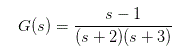Here one zero at s = 1, Two poles at s = - 2, s = - 3. Since zero lies in RHS of s - plane. It is nonminimum phase type, since both poles lie in LHS of s - plane, so the system is stable.

04․ A function y (t) satisfies the following differential equation :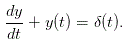Where, δ (t) is the delta function. Assuming zero initial condition and denoting the unit step function by u(t), y(t) can be formed as
et.
e-tu(t).
e-t.
etu(t).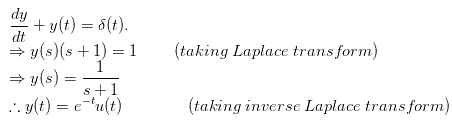05․ Lead network is used to
improve transient response.
both A and C.
increase bandwidth.

Lead network improves transient response and increases margin of stability and increases bandwidth.

06․ Type and order of transfer function G (s) = K / {s(s + 2)}
1, 2.
2, 1.
0, 2.
1, 1.

Type of a system is defined the total number of poles at the origin of the transfer function and order of a system is defined the highest power of ‘s’ in denominator. Here total number of poles located at the origin is 1, so type of the transfer function is 1. Here highest power of ‘s’ in denominator is 2. So, the order of the transfer function is 2.

07․ Name test signals used in time response analysis?
All of B, C, D.
Unit step.
Unit ramp.
Impulse.

All these signals are used in time response analysis.

08․ A system is stable for (where GM = Gain Margin and PM = Phase Margin):
GM and PM both +ve.
GM and PM both -ve.
GM -ve.
PM - ve.

For a system to be stable both GM (gain margin) and PM (phase margin) should be positive.

09․ Transfer function of zero order hold response is
(1 / s) × (1 - est).
(1 / s) × (1 - e-st).
(s) × (1 - est).
(1 / s) × e-st.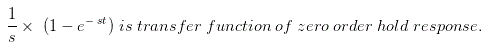10․ A linear time invariant system has an impulse response e2t for t > 0 . If initial condition are 0 and input is e3t, the output for t > 0 is
e2t - e3t.
e3t - e2t.
e2t + e3t.
e5t.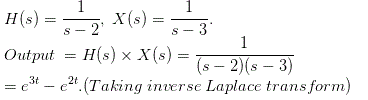<<<678910>>>